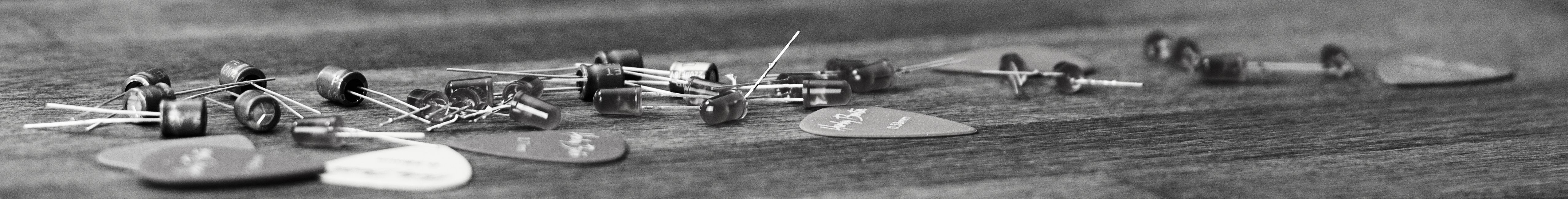savchenko.tech / software engineering blog by Maryna Savchenko## Why A Square Is Not A Rectangle: Liskov Substitution Principle

2023-02-19

Liskov substitution principle (LSP) is one of the five SOLID principles. It is based on the concept of “substitutability”. Principle allows using code to be written in terms of the supertype specification, yet work correctly when using objects of the subtype.

“What is wanted here is something like the following substitution property: If for each object o1 of type S there is an object o2 of type T such that for all programs P defined in terms of T, the behavior of P is unchanged when o1 is substituted for o2 then S is a subtype of T.1”

Barbara Liskov, 1988

In other words software systems should be built from substitutable (interchangeable) parts. If superclass does something, subclass should do it too (it should be substitutable for the superclass).

What Is The Problem With Rectangle Being Derived From Square?

Let’s create Rectangle and Square classes with calculateArea() method on parent Rectangle class.``````public class Rectangle {
protected double height;

protected double weight;

public Rectangle(double height, double weight) {
this.height = height;
this.weight = weight;
}

public double calculateArea() {
return height * weight;
}
}
``````
``````public class Square extends Rectangle {
public Square(double side) {
super(side, side);
}
}
``````

Tests for calculating area for both Rectangle and Square are green. No obvious problem so far.

``````@Test
void shouldCalculateRectangleArea() {
Rectangle rectangle = new Rectangle(2, 4);
double area = rectangle.calculateArea();

assertEquals(8, area);
}

@Test
void shouldCalculateSquareArea() {
Rectangle rectangle = new Square(4);
double area = rectangle.calculateArea();

assertEquals(16, area);
}
``````

The problem appears when there is a need to mutate separately height and weight.

This will make setters for height and weight available on Square also and can end up in incorrectly modified shape.

``````    @Test
void shouldSetWeightOfRectangle() {
Rectangle rectangle = new Rectangle(2, 4);
rectangle.setWeight(6);
assertEquals(2, rectangle.getHeight());
assertEquals(6, rectangle.getWeight());

}

@Test
void shouldSetSideOfSquare() {
Rectangle square = new Square(4);
square.setWeight(6);
// different values, should not be happening
assertEquals(6, square.getWeight());
assertEquals(4, square.getHeight());
}
``````

So Square subtype is not substitute Rectangle, because Square has fewer properties yet inherit access methods of super class Rectangle.

Of course, the problem can be solved with additional check on caller’s side. But this is not how we want to use polymorphism.

Violation of Liskov substitution principle can lead to pollution of the system with extra logic which will make code more fragile and less readable.

Implementation with tests can be found on GitHub.

Sources: